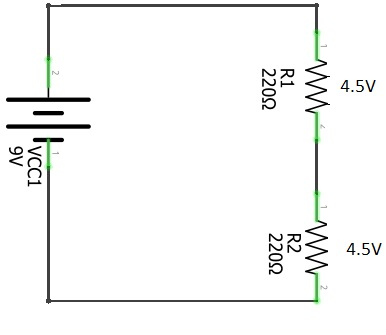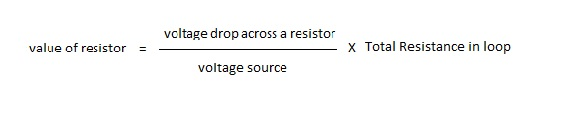# Using Resistors for Voltage division

Resistors as we know, if not an exaggeration are the most popular electronics components in history. They play so many vital roles in all electronic circuits from limiting currents and voltages to even aiding in amplification of currents and voltages.

In this piece we are going to learn how to use resistor for voltage division.

## What is voltage division?

Okay imagine you are building a circuit that is suppose to control 2 motors and 1 LED in a robot you are building. Your  voltage source is say 9V but each motor has a particular voltage it requires. Motor 1 needs 3volts, motor 2 needs 3.5volts, and the LED needs 2.5volts.

You can see that the voltage source is 9V but each load is requiring a unique voltage across it. You can’t connect the 9V to all the components just like that, you may end up frying delicate components. Hence, there has to be a way to divide a given voltage source to suit all the unique parts of your circuit. That is all what voltage division is about.

## The voltage divider circuit

The voltage division circuit is simple and is based on ohm’s law. In a closed loop consisting of a voltage source and two resistors of the same value in series,there will be equal voltage drop across the two resistors and this is proven to be true by kirchhoff’s voltage law which states that, “the algebraic sum of voltages in a closed loop is zero”. For details, this is the first concept you should know.

If the voltage source is 9V, and the resistors are of the same value, you will have 4.5V across both resistors. (9V-4.5V-4.5V=0V). In other words, the 9V has been divided into 2 equal parts. In case you have a components that requires 4.5V, you can just connect that component across any of the resistors(thus in parallel). This is a simple voltage divider concept.

In some cases you will want maybe 3 distinct voltages like in the example of the robot having 2 motors and 1 LED. In this case, you will need at least 3 different resistors each of different values. In a series connection, we know that the current passing through all the components are the same despite the value of the resistors. Nevertheless, the voltage drop across the resistors may differ depending on their individual resistances.

For example: If a same current of 15mA passes through three different resistors of values 100 ohms,200 ohms and 300 ohms.  The 100 ohm resistor will have 1.5V, the 200 ohm resistor will have 3V, and the 300 ohm resistor will have 4.5V across it. (V=IR).Note: with a voltage source of 9V.

From the circuit on the left, the three resistors have unknown resistances but at the end of the day, we want to have 3V across R1, 3.5V across R2 and 2.5V across R3. The voltage source is 9V.

This is what the voltage divider rule statesso to find the value of say resistor R1, you put the parameters in the above equation and make R1 the subject.

• R1=the unknown resistance
• 3V=voltage drop across R1
• 9v=total voltage of the circuit
• R1+R2+R3=total resistance of circuitPutting in the available parameters we have:Pay attention to the relation above. 3/9 is 1/3. Hence the value of R1 is supposed to be 1/3rd of whatever the total resistance of the circuit is. It is so with the rest of the Resistors.

“The unknown resistance is the product of (the total resistance) and (the ratio of voltage drop across resistor and total voltage).”

## Do some magic with the LDR

Overview Materials needed Set up Overview What you need to know; Ohms law. Voltage division. How to use the breadboard. How the LDR works. In…

## Potentiometer Controlled LED

Overview Materials Set up Overview In the “toggle an LED” article you realize that your circuit had only two distinct events, a HIGH (1) thus…

## Your Op-amp needs this power supply circuit

Hei there, welcome to another tutorial from Aaenics. Today we want to learn something special when it comes to working with Operational amplifiers. Its more…

## Toggle that LED HIGH

Overview Materials Set up Overview Getting  an LED toggled high is not a big deal until reality hits you unaware. Many students have theoretically constructed…

## The 555 timer IC.

As a hobbyist, engineer, or maybe an electronics geek or wherever you find yourself, you can’t really have the time and pleasure to know what goes on in all the million ICs in the world. However you can delve with me into this simple 555 timer to see whats going on in it. This can give you a certain level of understanding about almost all ICs.